#Function Repository Resource:

# FubiniNumber

Count the number of ways to partition a set where the order of the subsets matters

Contributed by: George Beck
 ResourceFunction["FubiniNumber"][set] counts the number of ways to partition set into subsets, where the order of the subsets matters.

## Examples

### Basic Examples

The simplest non-trivial Fubini number:

 In:=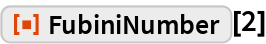Out=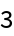Here are the first 10 Fubini numbers:

 In:=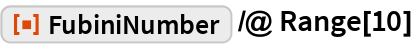Out=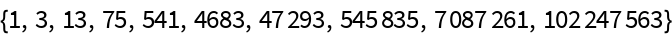### Scope

This shows the 13 ordered set partitions of the the set {1,2,3}:

 In:=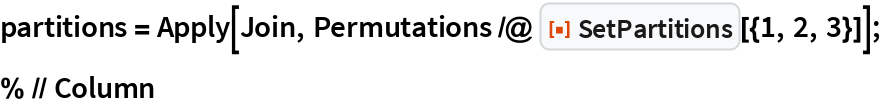Out=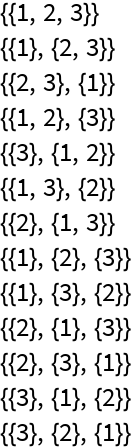In:=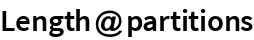Out=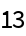This accords with:

 In:=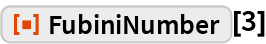Out=### Neat Examples

The Fubini numbers are periodic mod 10:

 In:=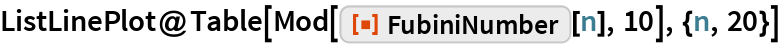Out=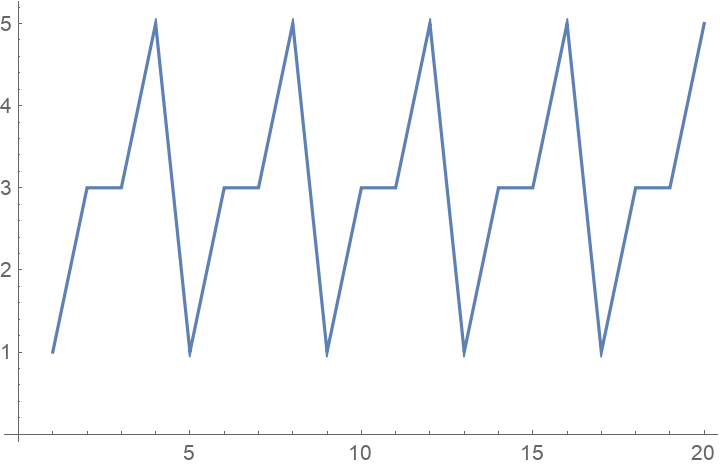There are many other modular relations:

 In:=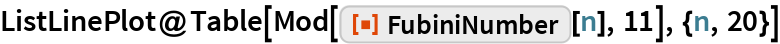Out=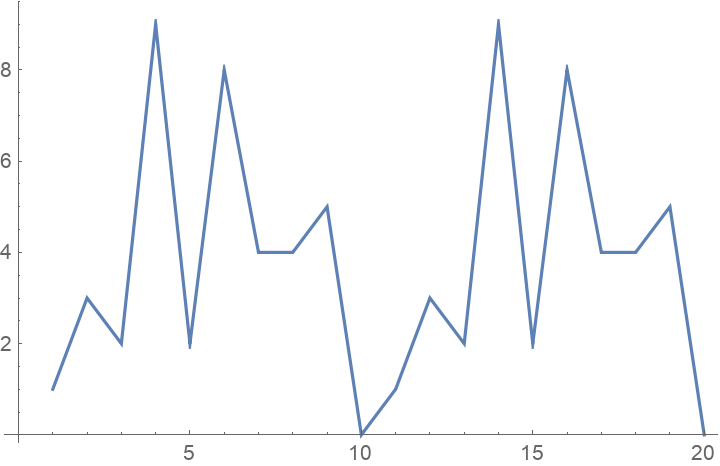## Requirements

Wolfram Language 11.3 (March 2018) or above